# RS Aggarwal Solutions for Class 6 Maths Chapter 2 Factors and Multiples Exercise 2E

RS Aggarwal Solutions for Class 6 Maths Chapter 2 Factors and Multiples Exercise 2E are available in PDF format. Exercise 2E consists of problems based on determining the LCM using the prime factorization method. RS Aggarwal Solutions contain well-structured solutions, which help students understand them quickly. The solutions are provided to students to solve the problems quickly from the exam point of view.

Faculty at BYJU’S has solved the RS Aggarwal textbook as per the latest CBSE syllabus. Students who practice these solutions will score high marks in the examinations. From Exercise 2E students learn the topics LCM effectively.

## Download PDF of RS Aggarwal Solutions for Class 6 Maths Chapter 2 Factors and Multiples Exercise 2E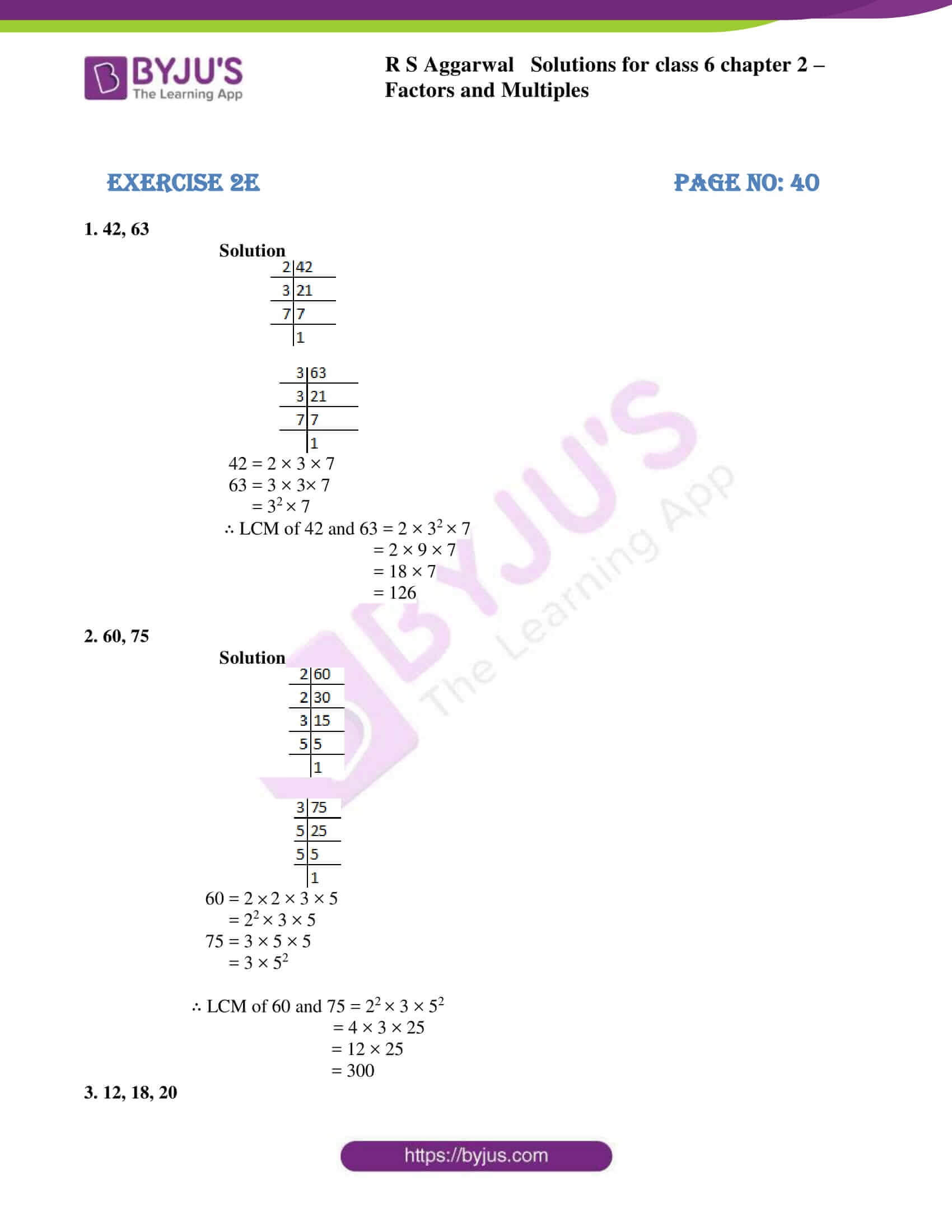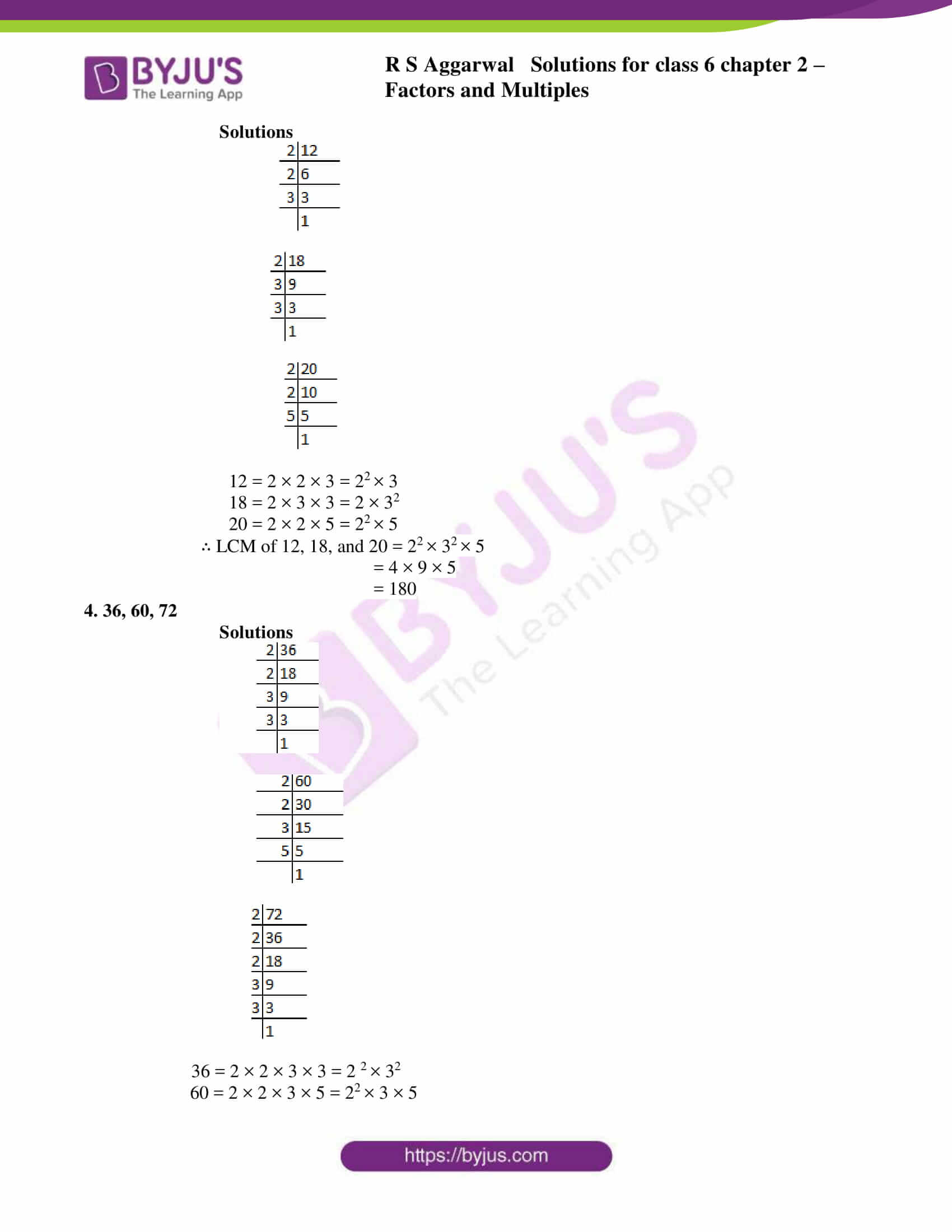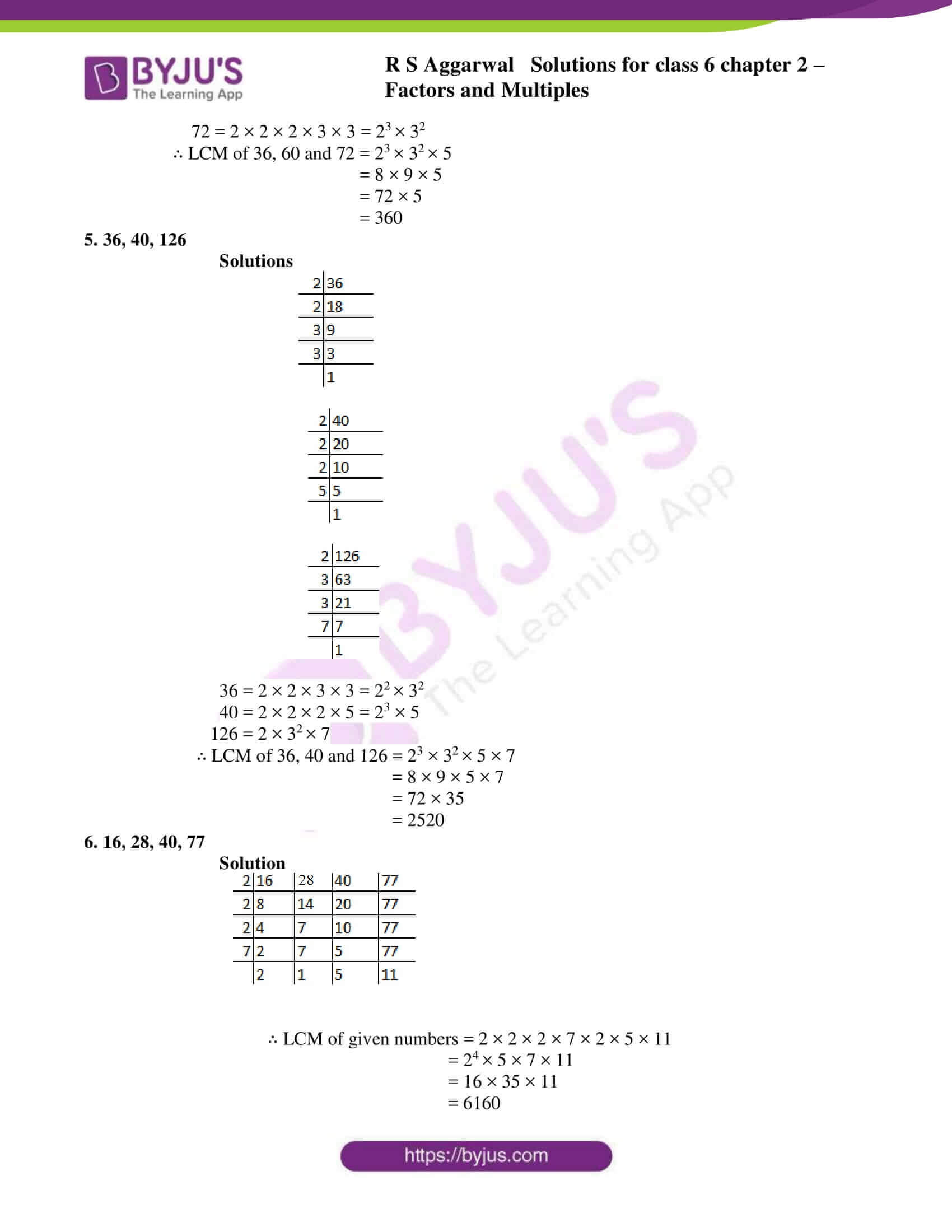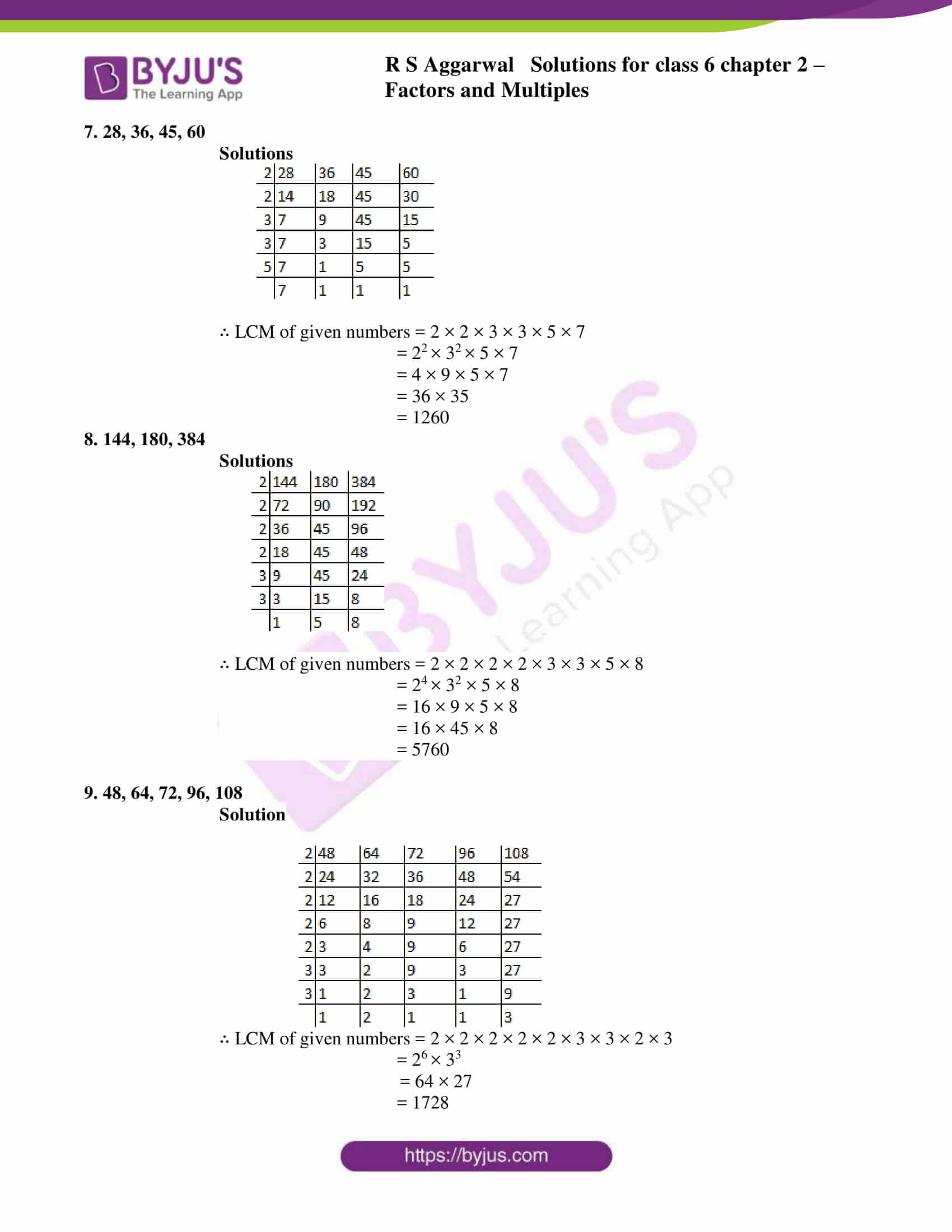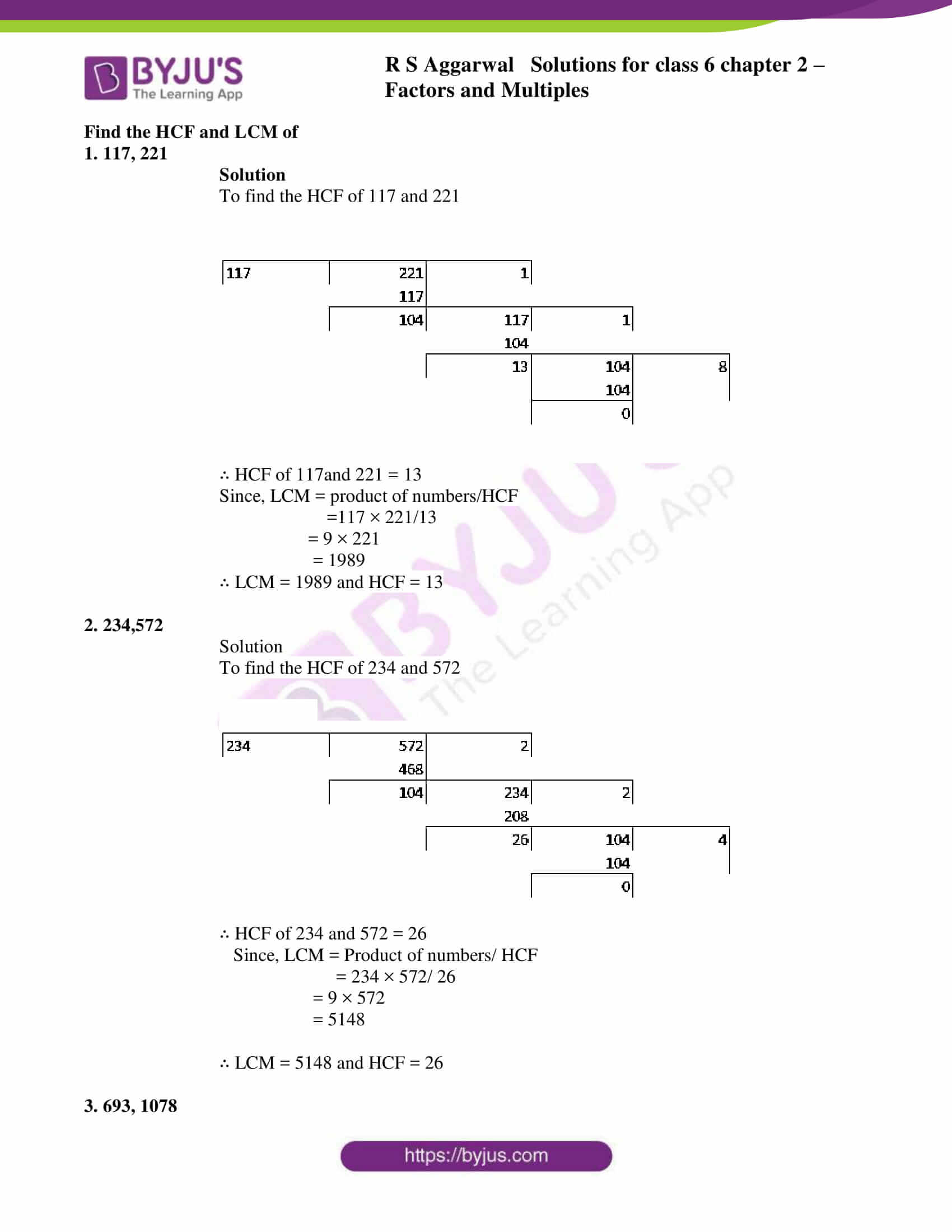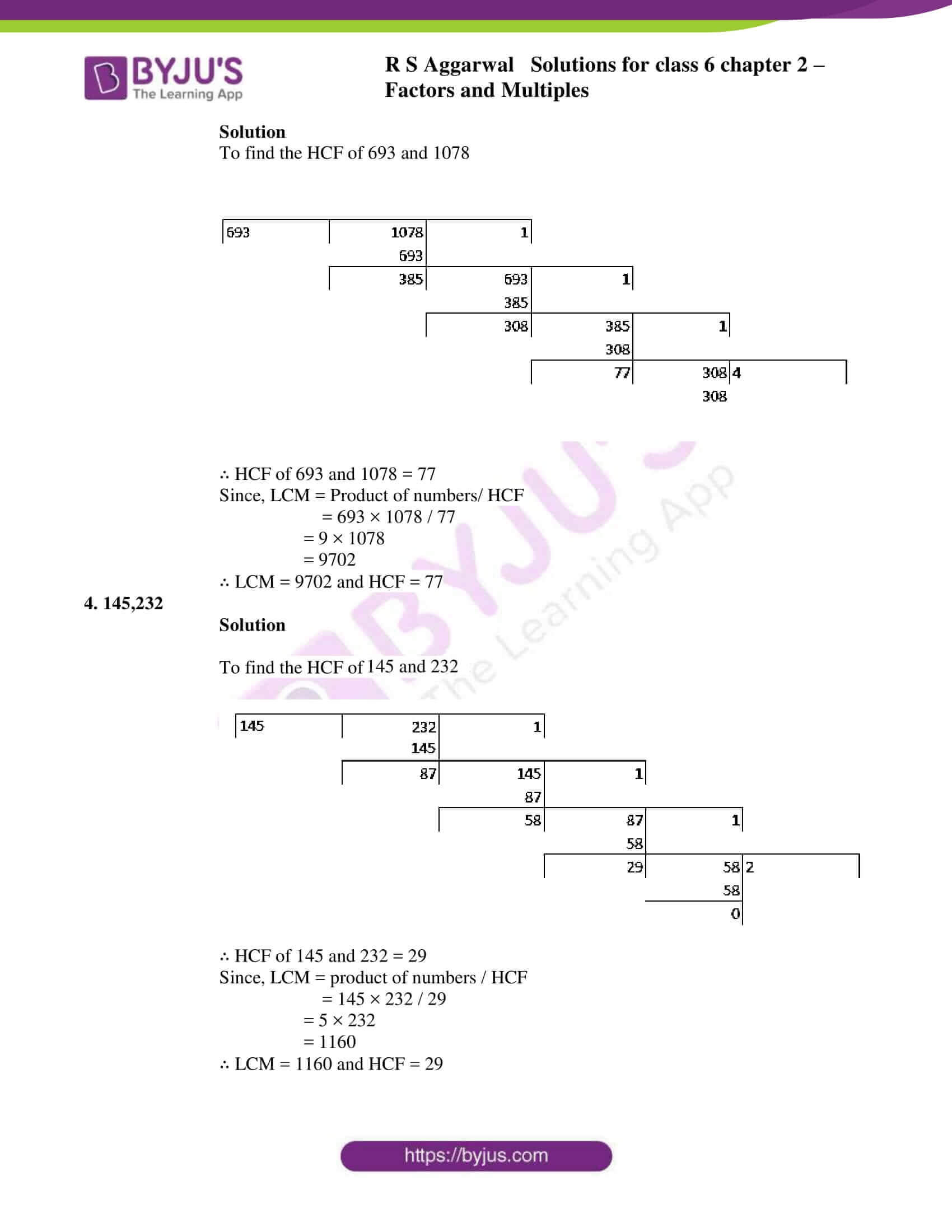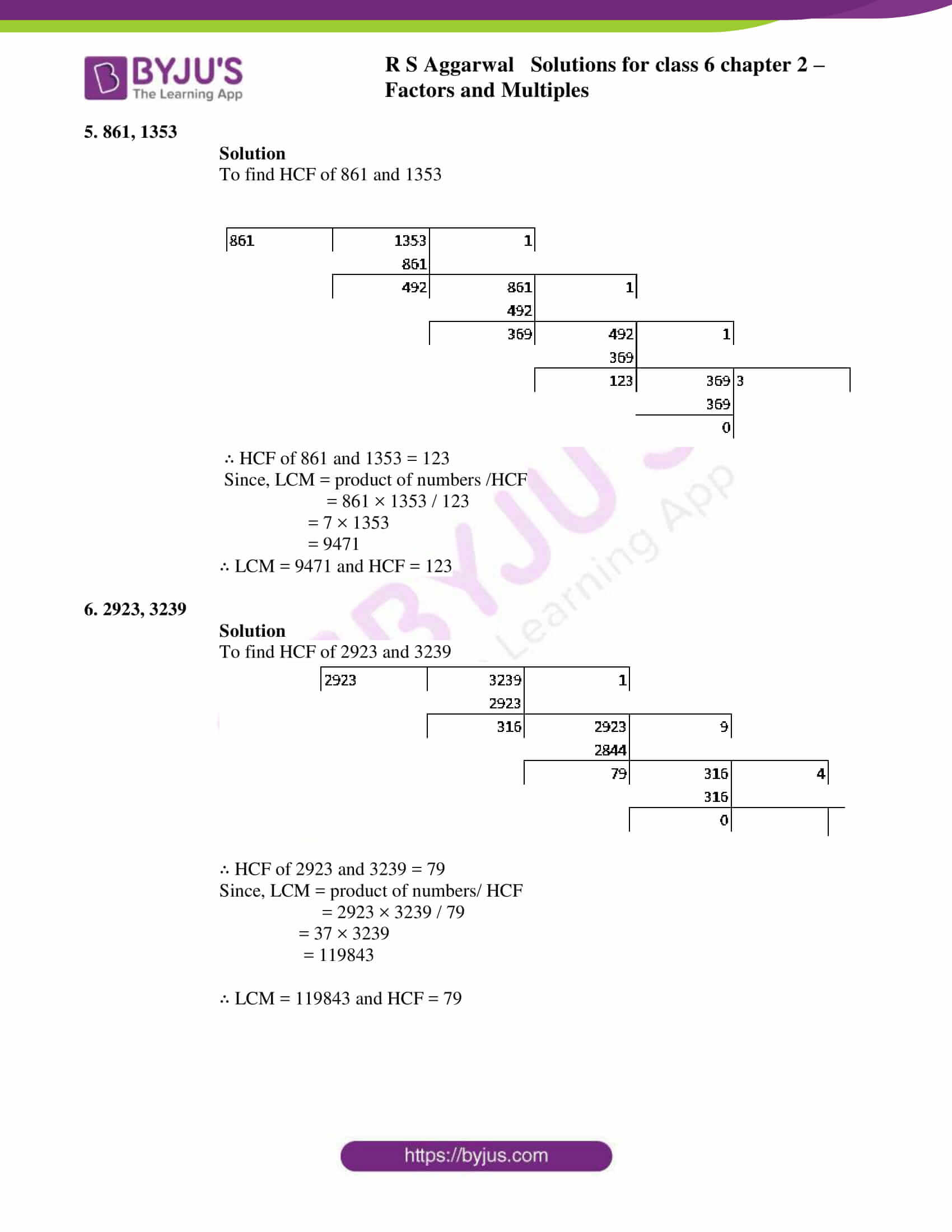### Access answers to Maths RS Aggarwal Solutions for Class 6 Chapter 2 Factors and Multiples Exercise 2E

1. 42, 63

Solution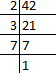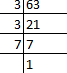42 = 2 × 3 × 7

63 = 3 × 3× 7

= 32 × 7

∴ LCM of 42 and 63 = 2 × 32 × 7

= 2 × 9 × 7

= 18 × 7

= 126

2. 60, 75

Solution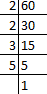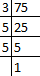60 = 2 × 2 × 3 × 5

= 22 × 3 × 5

75 = 3 × 5 × 5

= 3 × 52

∴ LCM of 60 and 75 = 22 × 3 × 52

= 4 × 3 × 25

= 12 × 25

= 300

3. 12, 18, 20

Solutions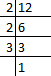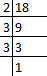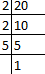12 = 2 × 2 × 3 = 22 × 3

18 = 2 × 3 × 3 = 2 × 32

20 = 2 × 2 × 5 = 22 × 5

∴ LCM of 12, 18, and 20 = 22 × 32 × 5

= 4 × 9 × 5

= 180

4. 36, 60, 72

Solutions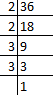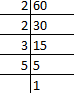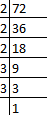36 = 2 × 2 × 3 × 3 = 2 2 × 32

60 = 2 × 2 × 3 × 5 = 22 × 3 × 5

72 = 2 × 2 × 2 × 3 × 3 = 23 × 32

∴ LCM of 36, 60 and 72 = 23 × 32 × 5

= 8 × 9 × 5

= 72 × 5

= 360

5. 36, 40, 126

Solutions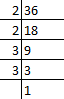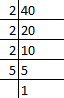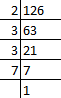36 = 2 × 2 × 3 × 3 = 22 × 32

40 = 2 × 2 × 2 × 5 = 23 × 5

126 = 2 × 32 × 7

∴ LCM of 36, 40 and 126 = 23 × 32 × 5 × 7

= 8 × 9 × 5 × 7

= 72 × 35

= 2520

6. 16, 28, 40, 77

Solution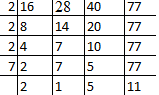∴ LCM of given numbers = 2 × 2 × 2 × 7 × 2 × 5 × 11

= 24 × 5 × 7 × 11

= 16 × 35 × 11

= 6160

7. 28, 36, 45, 60

Solutions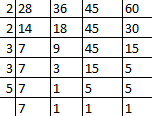∴ LCM of given numbers = 2 × 2 × 3 × 3 × 5 × 7

= 22 × 32 × 5 × 7

= 4 × 9 × 5 × 7

= 36 × 35

= 1260

8. 144, 180, 384

Solutions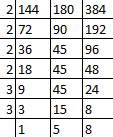∴ LCM of given numbers = 2 × 2 × 2 × 2 × 3 × 3 × 5 × 8

= 24 × 32 × 5 × 8

= 16 × 9 × 5 × 8

= 16 × 45 × 8

= 5760

9. 48, 64, 72, 96, 108

Solution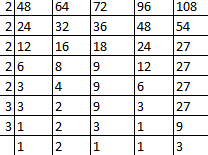∴ LCM of given numbers = 2 × 2 × 2 × 2 × 2 × 3 × 3 × 2 × 3

= 26 × 33

= 64 × 27

= 1728

Find the HCF and LCM of

1. 117, 221

Solution

To find the HCF of 117 and 221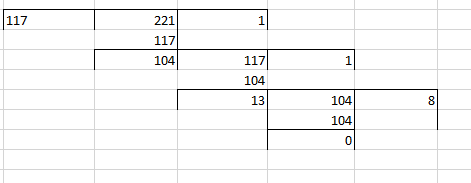∴ HCF of 117and 221 = 13

Since, LCM = product of numbers/HCF

=117 × 221/13

= 9 × 221

= 1989

∴ LCM = 1989 and HCF = 13

2. 234,572

Solution

To find the HCF of 234 and 572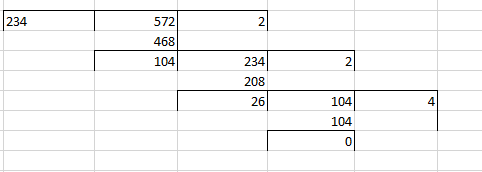∴ HCF of 234 and 572 = 26

Since, LCM = Product of numbers/ HCF

= 234 × 572/ 26

= 9 × 572

= 5148

∴ LCM = 5148 and HCF = 26

3. 693, 1078

Solution

To find the HCF of 693 and 1078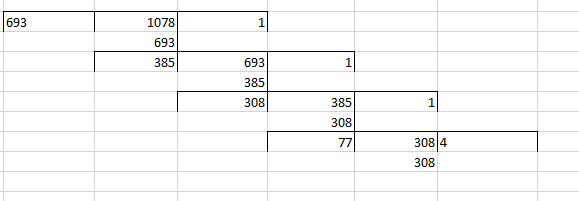∴ HCF of 693 and 1078 = 77

Since, LCM = Product of numbers/ HCF

= 693 × 1078 / 77

= 9 × 1078

= 9702

∴ LCM = 9702 and HCF = 77

4. 145,232

Solution

To find the HCF of 145 and 232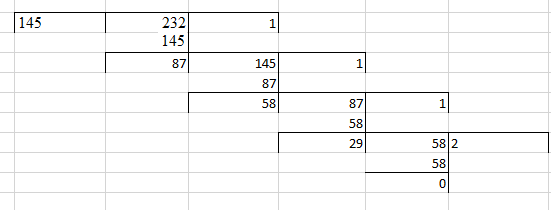∴ HCF of 145 and 232 = 29

Since, LCM = product of numbers / HCF

= 145 × 232 / 29

= 5 × 232

= 1160

∴ LCM = 1160 and HCF = 29

5. 861, 1353

Solution

To find HCF of 861 and 1353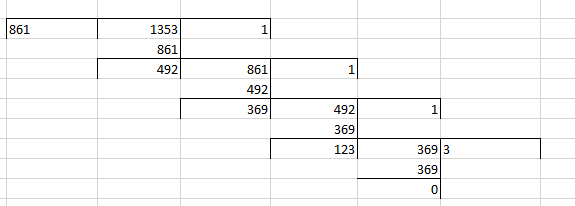∴ HCF of 861 and 1353 = 123

Since, LCM = product of numbers /HCF

= 861 × 1353 / 123

= 7 × 1353

= 9471

∴ LCM = 9471 and HCF = 123

6. 2923, 3239

Solution

To find HCF of 2923 and 3239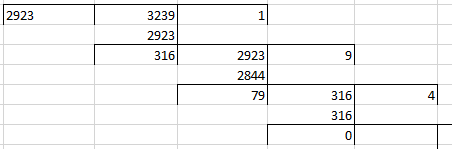∴ HCF of 2923 and 3239 = 79

Since, LCM = product of numbers/ HCF

= 2923 × 3239 / 79

= 37 × 3239

= 119843

∴ LCM = 119843 and HCF = 79

## RS Aggarwal Solutions for Class 6 Maths Chapter 2 Factors and Multiples Exercise 2E

RS Aggarwal Solutions for Class 6 Maths Chapter 2 Factors and Multiples Exercise 2E are based on LCM of given numbers and finding them using prime factorization method. Students can gain expertise in solving problems related to LCM using RS Aggarwal Solutions for Class 6 Maths Chapter 2 Factors and Multiples Exercise 2E. Topics present in other exercises are

• Finding prime and composite numbers
• General properties of divisibility
• Prime factorization
• To find HCF

RS Aggarwal Solutions provide step wise solutions for students which is easy for practice. It helps them score good marks and also to enhance their knowledge about concepts related to the subject.